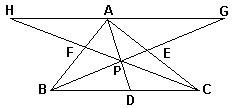# Van Obel Theorem and Barycentric coordinates

The Van Obel theorem may serve as a link between Ceva's Theorem and barycentric coordinates. (Another proof of Van Obel's theorem builds on the ratios of areas formed by concurrent cevians in a triangle.)

### Theorem

For three cevians meeting inside ΔABC at point P, the following is true:(1) AP/PD = AE/EC + AF/FB

From the mechanical point of view, van Obel's theorem asserts a recombination property of the center of mass. The center of mass of three points can be computed in two ways: first, as the average of the three points and the location of the cumulative mass. Second, as the center of mass of one of the three points (A in the diagram) and the center of mass of the other two (B and C).

The proof is very simple and follows easily from juxtaposition of three pairs of similar triangles: PHG and PBC, AEG and EBC, and AFH and BCF.

For the following I just have to simplify notations. Which actually means introducing a lot of new symbols. So, let u = PD/AD, v = EP/EB, w = FP/FC, and CD/DB = ra, AE/EC = rb, BF/FA = rc. Ceva's theorem says that

 (2) rarbrc = 1.

Van Obel's theorem is equivalent to 1/u = rb + 1/rc + 1. Which, from (2), is the same as

 (3) 1/u = rb + rarb + 1

Similarly,

 (4) 1/v = rc + 1/ra + 1 = rc + rcrb + rarbrc = rc(1 + rb + rarb)

and

 (5) 1/w = ra + 1/rb + 1 = ra + rarc + rarbrc = ra(1 + rc + rbrc) = ra(rarbrc + rc + rbrc) = rarc(1 + rb + rarb)

Therefore

 (6) u + v + w = (1 + 1/rc + rb)/(1 + rb + rarb)

With one additional application of the life saving (2), the latter simplifies to u + v + w = 1, as expected. Are these the barycentric coordinates of point P? Yes, they are. In (3)-(5), u, v, and w are connected exactly in the manner described at the end of the discussion on Ceva's Theorem; so that u = wA, v = wB, and w = wC. Also note that from the definition u = PD/AD. It follows that u = area(ΔPBC)/area(ΔABC) and similarly for v and w. The barycentric coordinates of a point P inside ΔABC are proportional to the areas of triangles P forms with the three sides of the triangle.### Barycenter and Barycentric Coordinates